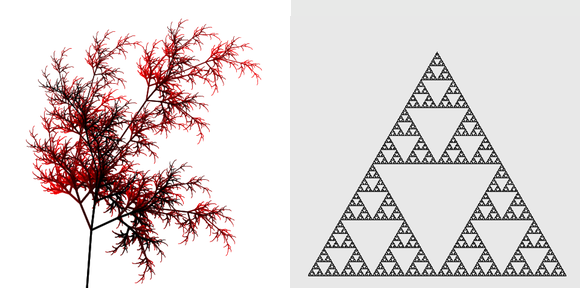# Simple L‑system generation

As I learn more about programming, I like to occasionally revisit problems I have implemented solutions for in the past, to see if I can produce a better implementation. A couple of years ago I implemented a simple L-system generator and evaluator with a turtle in Javascript + Canvas. I was recently reminded of this by moogatronic on the `#clojure` IRC channel, and decided to see what a Clojure solution might look like.L-systems are an interesting use of generative grammars to describe complex recursive structures. They are frequently discussed as a tool for generating of fractal graphics, but are also useful for generating procedural music1. I’ll be using the Sierpinksi Triangle as an example later in this article.

Before we look at some code, lets briefly examine the basic components of an L-system:

Productions
A production is a rule mapping a symbol to the sequence of symbols that replaces it.
Symbol
A symbol is an element of the string. The exact meaning of the symbol is not important during generation, but is useful when evaluating the resulting structure. There are two types of symbol: Non-terminals and constants. A constant is simply a symbols whose production is simply itself; an non-terminal is any other kind of production.
Start
This is the symbol to start evaluation from.

To represent this, a production is going to be a mapping from some type to a sequence of that type. This might be a keyword to a vector of keywords or a character to a string of characters. E.g, we could define the Algea rules as one of the following:

``````{\A "AB", \B "A"}           ; using characters and strings
{:A [:A :B], :B [:A] }      ; using keywords and vectors
``````

In this case we have only non-terminals and no constants. The following function is all that is needed to take a set of rules like the above and produce a full structure to a given depth:

``````(defn l-system
[productions depth s]
(if (zero? depth) s
(mapcat #(l-system productions (dec depth) (productions % [%])) s)))
``````

And an example of its use:

``````(l-system {\A "AB" \B "A"} 3 "A")
; => (\A \B \A \A \B)
``````

As you can see, this produces the same results given in the wikipedia page. Note that the starting symbol in this case is actually provided in a sequence; This is to satisfy the recursive definition of `l-system`.

What makes this implementation so simple is the recursive application of `mapcat` with the productions. This exactly maps onto the conceptual model of what an L-system is.

The particular `mapcat` function argument is slightly more complex than purely the productions; This is responsible for three things:

• Recursing if there is remaining depth
• Handling the difference between non-terminals and constants.

This later case is handled with the slightly curious form:

``````(productions % [%])
``````

This uses the associative access interface in Clojure to supply a key and a default value. The default value is a sequence containing just the key. In other words if there is no production for a symbol, then just generates the symbol itself2.

By themselves these structures are not very interesting; for one, there is no semantic meaning to any of the symbols. The particulars of how the resulting structure is produced is dependent on what you want to do with it. The following function takes a map of functions that operate on an (notionally) immutable turtle:

``````(defn run-turtle
[turtle operations commands]
(reduce (fn [t c] ((operations c identity) t)) turtle commands))
``````

If you knew you were dealing with a mutable turtle (such as the primitive Java2d one below) you might opt to use a different `run-turtle` implementation (using `doseq` for example).

The next two blocks of code are provided for completeness rather than elegance:

``````(import (javax.swing JFrame JPanel)
(java.awt Graphics2D RenderingHints)
(java.awt.image BufferedImage))

(defn ^Graphics2D create-turtle [x y]
(let [panel (JPanel.)
frame (doto (JFrame.)
(.setSize x y)
(.show))]
(doto (.getGraphics panel)
(.setRenderingHints
{RenderingHints/KEY_ANTIALIASING RenderingHints/VALUE_ANTIALIAS_ON}))))
``````

The following two operations are all we need for the Sierpinksi Triangle. For more complex L-systems, such as the iconic fern or trees, you will want more complex turtles and additional operations to manage the stack of context. Finally, both these functions return the turtle so that they can run through the immutable `run-turtle` provided above.

``````(defn forward
[distance turtle]
(.drawLine turtle 0 0 0 distance)
(doto turtle
(.translate 0 distance)))

(defn rotate
[theta turtle]
(doto turtle
(.rotate theta)))
``````

You will notice that both these take the turtle as the last argument. This is so that they can be trivially specialized using `partial` for a particular operation as you will see below.

With the java2d code out of the way, here is a Sierpinksi Triangle implementation based on the grammar provided on the wikipedia page:

``````(defn sierpinski-triangle
[]
(let [rules      {\a "b-a-b"
\b "a+b+a"}
start      "a"
angle      (* 2 Math/PI (/ 60 360))
operations {\a (partial forward 1)
\b (partial forward 1)
\- (partial rotate (- angle))
\+ (partial rotate angle)}
system     (l-system rules 9 start)]
(run-turtle (doto (create-turtle 600 600)
(.translate 50 550)
(.rotate (/ Math/PI -2)))
operations
system)))
``````

By now you should be aware of two things: `l-system` is a nice function, and my turtle code is rubbish.

You might find my old javascript implementation interesting for comparison. I also have a javascript implementation of the above code as a gist.

1. Moogatronic was planning to use his own L-system generator with a Clojure project called Overtone.
2. Note that while `productions` has been a map here, any function satisfying that interface would be acceptable. This might prove useful if you chose to implement a non deterministic grammar.

9 November 2011